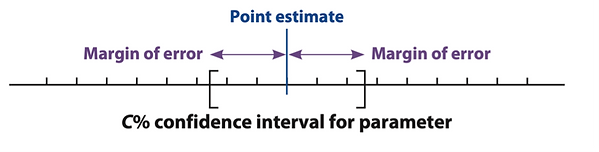top of page

## Chapter 8 - Day 1

##### Learning Targets
• Interpret a confidence interval.

• Determine the point estimate and margin of error from a confidence interval.

• Use confidence intervals to make decisions.

##### Sample Chapter 7 Test Scores

Experience First

In this lesson, students will try to guess the mystery mean (the mean of the Chapter 7 Test scores) by taking a random sample of 5 students. We suggest writing each of the test scores on a piece of paper and putting them in a paper bag. We have provided a sample of Chapter 7 test scores if you don’t want to use class data for your students.

In question #5, students will be choosing a value for their margin of error that will allow them to be 95% confident that their interval captures the true mystery mean. Challenge them to lower this value and see what happens to their confidence. After they complete question #6, ask them if they can “come up with a formula for making a confidence interval.”

Formalize Later

When debriefing the activity, try to make it clear to students that the reason we need a margin of error is because of sampling variability – every random sample produces slightly different results. Therefore, our sample mean is not likely to be exactly equal to the mystery mean, so we use a margin of error instead to create an interval of plausible values (plausible = believable). Many people incorrectly assume that “margin of error” is needed because of some possible “errors” in the data collection process. Maybe we should

At the end of the debrief of the activity,be sure to reveal that actual value for the Mystery Mean. You can then ask students to check if the confidence interval they created does or does not contain this value.

Notice in the QuickNotes that we are providing a template that students can use for interpreting confidence intervals. We have found this strategy to be very helpful for our English Language Learners and you will see several more instances of this in later lessons in this Chapter.

For visual learners, show them this helpful image for understanding how to build a confidence interval. Understanding this image will also be helpful for students success in the next lesson.bottom of page